Yashb | Convert image to text using PYTHON!

# Convert image to text using PYTHON!

Abdallah Alaa Alwarawreh 17 Feb, 2022 3 min

Today we will be making a python program that takes in an image and outputs it as black and white text.

This is how the end result looks: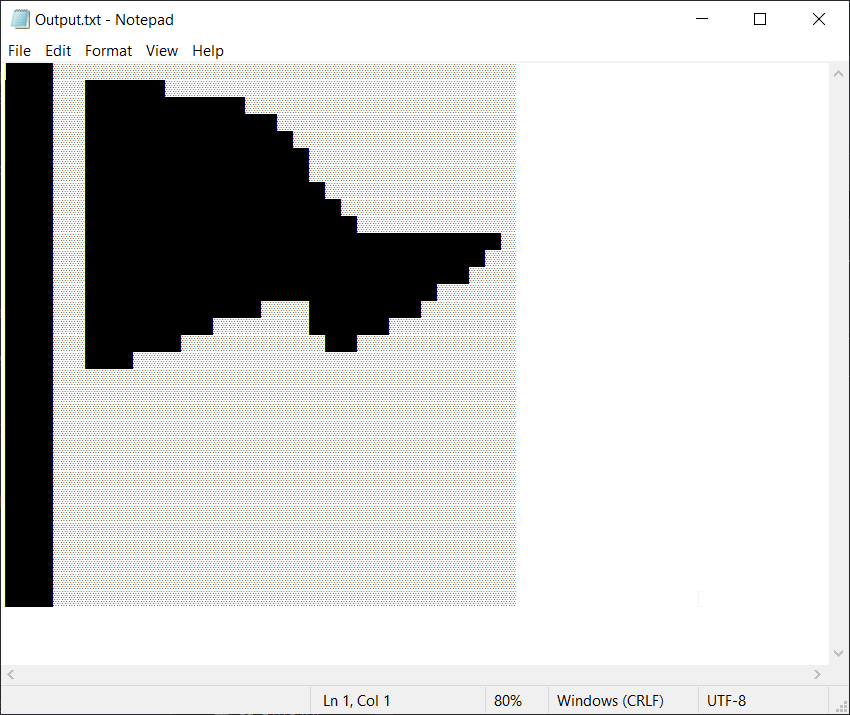This is what the project structure looks like:

• ImageToText
• Main.py
• Flag.png
• Output.txt

Required packages are:

Install opencv-python using pip:

• pip install opencv-python

With all of that out of the way, let's get into the code.

We need to import the OpenCV library

```import cv2
```

Now we need to open an image we can use cv2.imread() like this:

```img = cv2.imread("flag.png")
```

In my case, I’m using flag.png as my image.

Now let's show the image on the screen:

```cv2.imshow("Flag", img)
cv2.waitKey(0)
```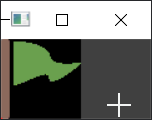Ok, nice, now let's convert the image to grayscale:

```img = cv2.cvtColor(img, cv2.COLOR_BGR2GRAY)
```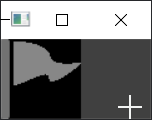Now after we converted it to grayscale, let’s make it black and white:

```ret, img = cv2.threshold(img, 80, 255, cv2.THRESH_BINARY)
```

Note: Change the number 80 to fit your needs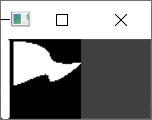Now into the fun part, converting the image to text, I went ahead and made 2 variables for black pixels and white pixels:

```Black = "░"
White = "█"
```

Now we need to loop through each pixel in the image like this:

```for i in range(img.shape):
for j in range(img.shape):
```

Now let's check if the pixel is black or white:

```if img[i][j] == 255:
FullWord+=White+White
else:
FullWord+=Black+Black
```

And after the second loop finish we add a new line like this:

```FullWord += "\n"
```

After we go through each pixel we write the “FullWord” into Output.txt:

```with open("Output.txt", "w", encoding="utf-8") as f:
f.write(FullWord)
```

Lets now run the code and see the output: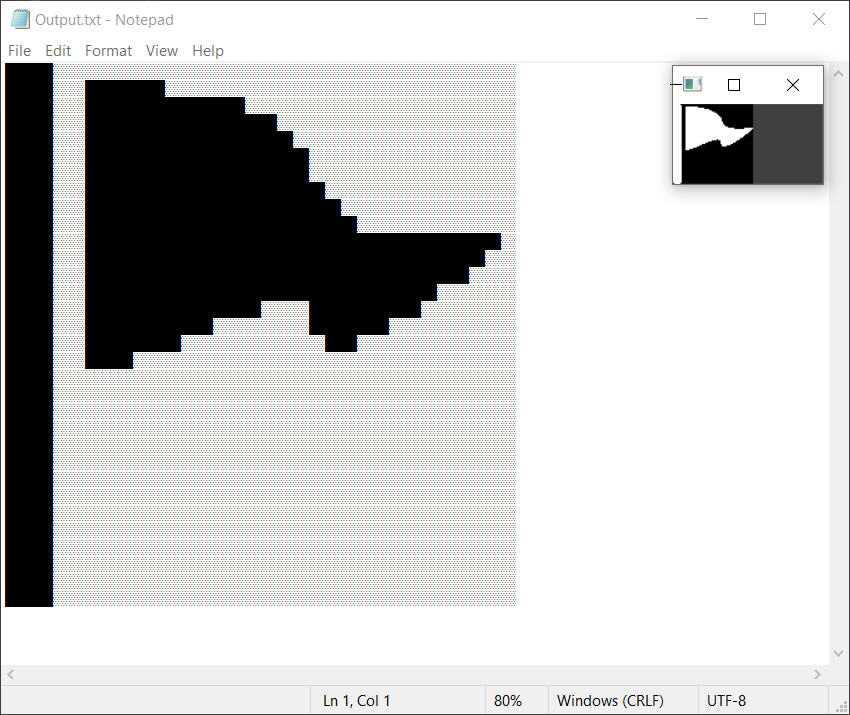Full Script:

```import cv2

img = cv2.cvtColor(img, cv2.COLOR_BGR2GRAY)
ret, img = cv2.threshold(img, 80, 255, cv2.THRESH_BINARY)
cv2.imshow("Flag", img)
cv2.waitKey(0)

Black = "░"
White = "█"

FullWord = ""
for i in range(img.shape):

for j in range(img.shape):
if img[i][j] == 255:
FullWord+=White+White
else:
FullWord+=Black+Black

FullWord += "\n"

with open("Output.txt", "w", encoding="utf-8") as f:
f.write(FullWord)

```